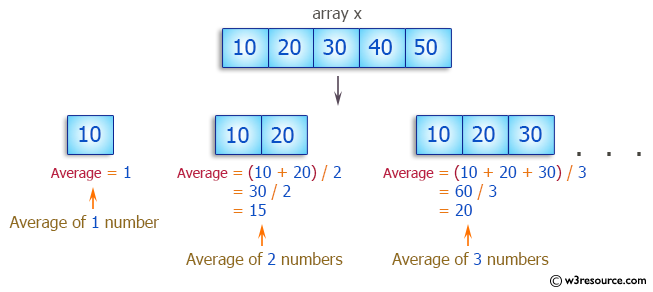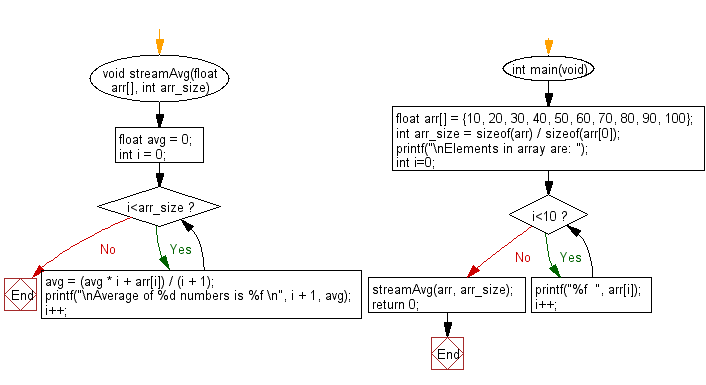﻿ C Program: Calculate the average of a stream of numbers

# C Exercises: Calculate and print average of the stream of given numbers

## C Programming Mathematics: Exercise-21 with Solution

Write a C program to calculate and print the average (or mean) of a stream of given numbers.

Example 1:
Input:
arr[] = {10, 20, 30, 40, 50, 60, 70, 80, 90, 100}
Output:
Average of 1 numbers is 10.000000
Average of 2 numbers is 15.000000
Average of 3 numbers is 20.000000
Average of 4 numbers is 25.000000
Average of 5 numbers is 30.000000
Average of 6 numbers is 35.000000
Average of 7 numbers is 40.000000
Average of 8 numbers is 45.000000
Average of 9 numbers is 50.000000
Average of 10 numbers is 55.000000

Pictorial Presentation:Sample Solution:

C Code:

``````#include <stdio.h>
#include <stdlib.h>

void streamAvg(float arr[], int arr_size)
{
float avg = 0;
int i = 0;
while(i<arr_size)
{
avg = (avg * i + arr[i]) / (i + 1);
printf("\nAverage of %d numbers is %f \n", i + 1, avg);
i++;
}
return;
}

int main(void)
{
float arr[] = {10, 20, 30, 40, 50, 60, 70, 80, 90, 100};
int arr_size = sizeof(arr) / sizeof(arr);
printf("\nElements in array are: ");
int i=0;
while(i<10)
{
printf("%f  ", arr[i]);
i++;
}
streamAvg(arr, arr_size);
return 0;
}
```
```

Sample Output:

```Elements in array are: 10.000000  20.000000  30.000000  40.000000  50.000000  60.000000  70.000000  80.000000  90.000000  100.000000
Average of 1 numbers is 10.000000

Average of 2 numbers is 15.000000

Average of 3 numbers is 20.000000

Average of 4 numbers is 25.000000

Average of 5 numbers is 30.000000

Average of 6 numbers is 35.000000

Average of 7 numbers is 40.000000

Average of 8 numbers is 45.000000

Average of 9 numbers is 50.000000

Average of 10 numbers is 55.000000
```

Flowchart:C Programming Code Editor:

Improve this sample solution and post your code through Disqus.

What is the difficulty level of this exercise?

Test your Programming skills with w3resource's quiz.

﻿